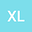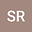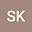Strong coupled fixed point analysis in fuzzy metric spaces and an application to Urysohn integral equations
•••• +3
• Xiangling Li,
• Saif Rehman,
• Sami Khan,
• Nawab Hussain,
• Hassen Aydi
Xiangling Li
Hebei University of Architecture

Corresponding Author:[email protected]

Author ProfileSaif Rehman
Gomal University
Author ProfileSami Khan
Gomal University
Author ProfileNawab Hussain
King Abdulaziz University
Author ProfileThe aim of this paper is to establish some strong coupled fixed point theorems via a new concept of cyclic contractive type mappings in the context of fuzzy metric spaces. Moreover, we ensure the existence of a common solution of the two Urysohn type integral equations:% for our result to get the existence theorem for common solution. The two Urysohn type integral equations are \begin{align*} &\xi(l)=\int_{a}^{b}K_1(l,s,\xi(s))ds+h_1(l),\\ &\xi(l)=\int_{a}^{b}K_2(l,s,\xi(s))ds+h_2(l), \end{align*} where $l\in[a,b]\subset\mathbb{R}$, $\xi,h_1,h_2\in C([a,b],\mathbb{R})$ and $K_1,K_2:[a,b]^2\times \mathbb{R}\to\mathbb{R}$